# Plug im!### Open access software for signal and image processing (http://www.plugim.fr/)

IFPEN provides its internal signal and image processing platform for those who want the most advanced features combined with a simple and understandable user interface.
The work of the thesis "Efficient morphological characterization of materials using distance transforms" (numerical descriptors of microstructures) are already available, or will be soon, as plug-ins in plug im!.
The developed plug-ins, directly linked to publications, as well as other digital operators, are detailed below. All of them are available under plug im!, as well as many other modules for signals, images and/or volumes processing.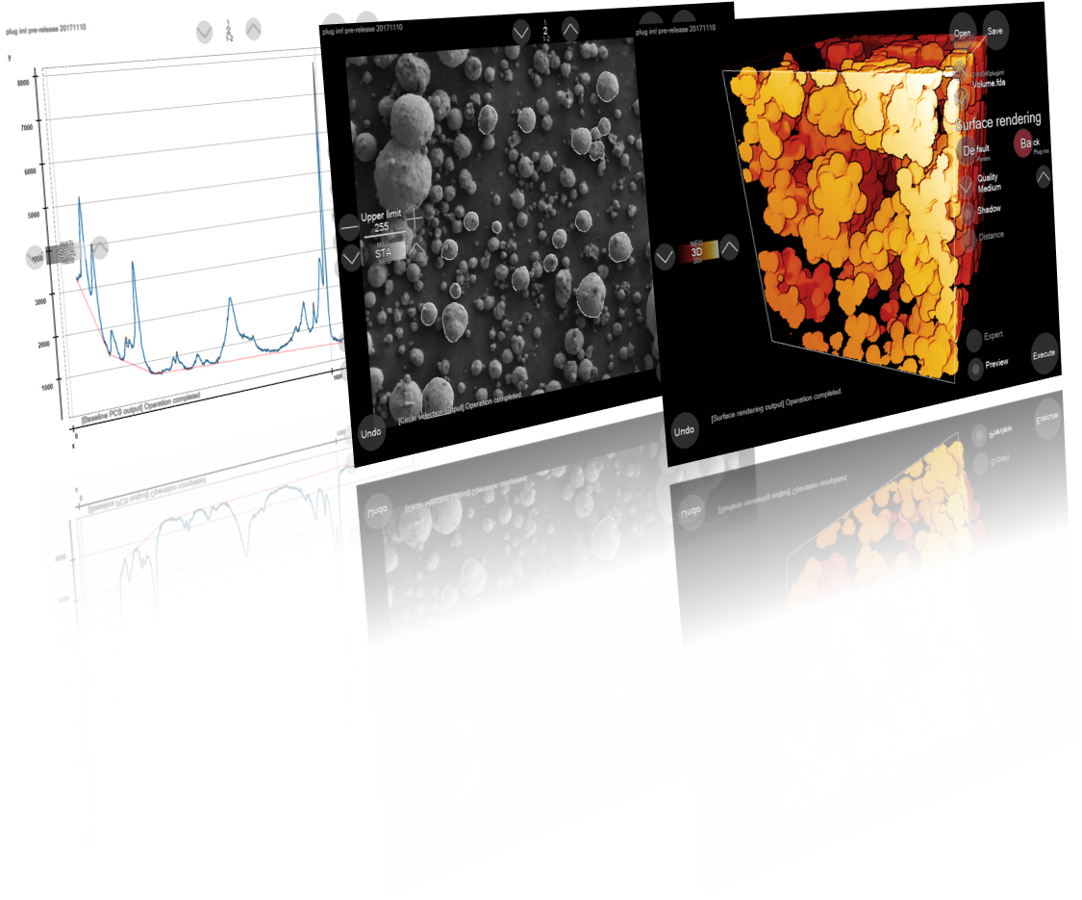Below, some plug-ins available under plug im! are detailed; the plug-in is briefly defined, then a help is given for its use (do not hesitate to contact me for any questions and/or remarks).
Plug-ins can be modified for various reasons: debugging, optimization, adding parameters ... The latest modifications will be indicated in bold.

...

## Available:

### H-tortuosity: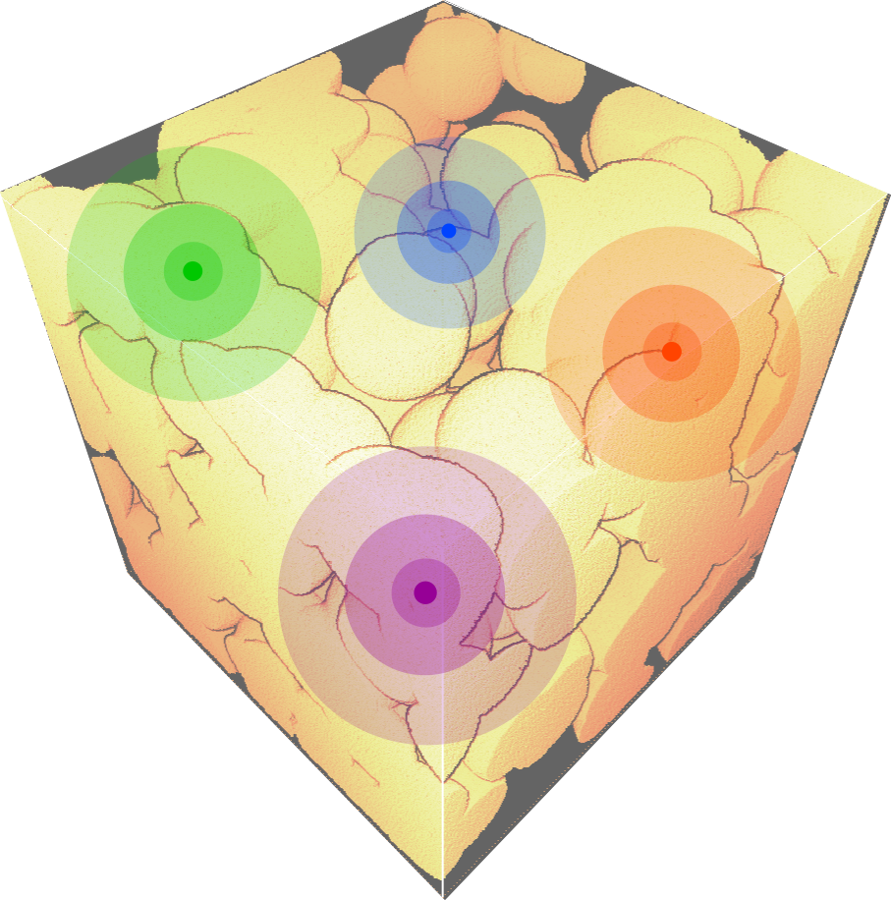Input:

• a binary 2D or 3D image, the white part represents the microstructure to analysis, OR a triphasic 2D or 3D image, with specific intensities: 0 for the surrounding void, meaningless, 255 for the solid phase, and the rest is considered as the porous phase.
The triphasic image can be the output of the plug-in "Extraction of porosity". This option is originally added to consider real images acquired with a specific device.

Outputs:

• the H-tortuosity values, the H-scalars
• the 2D or 3D map of the mean relative tortuosities.

Optional outputs:

• the 2D or 3D morphological tortuosities map for each starting point.

Parameters:

• the sampling method choice (1D-sampling or stratified-sampling),
• the maximal distance value Dmax (tortuosity variations assessed from 1 to Dmax),
• the number of random points (N),
• a boolean 'Save Data', to save the morphological tortuosities map of each starting point as *.fda file. Additional parameter (if checked):
1. "...': selection of the save directory.

Computation of the H-tortuosity estimator: a scalable topological descriptor providing a 3D map of mean relative tortuosities, a set of H-coefficients, each one for a given starting point and scale, of which the histograms could be meaningful, and a final curve, the H-tortuosity values, named the H-scalars, as a function of the scale, assessing the average variations of the peripheral morphological tortuosity.
The descriptor, based on a Monte Carlo process, handles disconnections and is applicable on complex multi-scale microstructures, especially when entries and exits are delicate to imposed. The various steps are similar to the M-tortuosity estimator.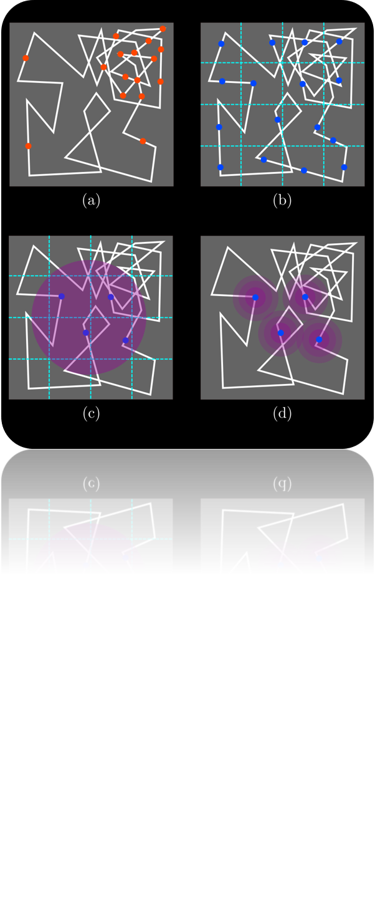1. Optional step: An optional step is the skeletonization. We recommand the computation of an homotopic skeleton of the interconnected microstructure to analysis. The homotopic skeleton is theoretically the smallest subset of the microstructure keeping the connectivity degree. In other words, the topology is unchanged. An exemple is shown in right figure.
Various computational methods are proposed in the state-of-the-art. Plug-ins will be available for the skeletonization step, different algorithms will be proposed according to the application, i.e. the nature of the image.
2. Sampling: The first step is the sampling of a chosen number of points N. Either N is the exact number of sampled points, if the 1D-sampling method (a) is chosen, or N is an approximated value, if the stratified-sampling method (b) is chosen. The next figure displays the difference the two sampling methods (a) and (b). An additional sub-step is imposed, especially in the case of stochastic models where different realizations are averaged. In order words, to avoid boundary issues, a sampling area is imposed, as displayed in the right figure (c).
3. Distance transforms computation: The distance transforms and the geodesic distance transforms are computed. At this step, the 2D or 3D map of mean relative tortuosities is assessed.
4. H-coefficients: As for the M-tortuosity, the H-coefficients can be seen as a set of morphological tortuosities, each one according to a specific random point, in other words " a viewpoint " of the microstructure of interest. Contrarily to the M-tortuosity, a H-coefficient is not only linked to a location, i.e. a point, but is linked to a scale too, i.e. a Euclidean distance, as shown in figure (d). Consequently, the computational process has to be adapted.
A H-coefficient, connected to a specific location and a given scale, is the assessed tortuosity at this point and this distance. It can be seen as either a viewpoint of the microstructure from this location and at this scale, or the average accessibility of this point for any random point of the microstructure located at the given distance from the current point.
5. H-scalars: The H-tortuosity values, named the H-scalars, is a set of viewpoints according to a given scale. Contrarily to the M-tortuosity which provides a global viewpoint of the overall microstructure, the H-tortuosity focuses on local features by quantifying them thanks to the local variations of the morphological tortuosity.
A H-scalar, connected to a specific scale, is the assessed average tortuosity of a pair of random points with the given distance as only constraint.

### H-tortuosity-by-iterative-erosions:

Input: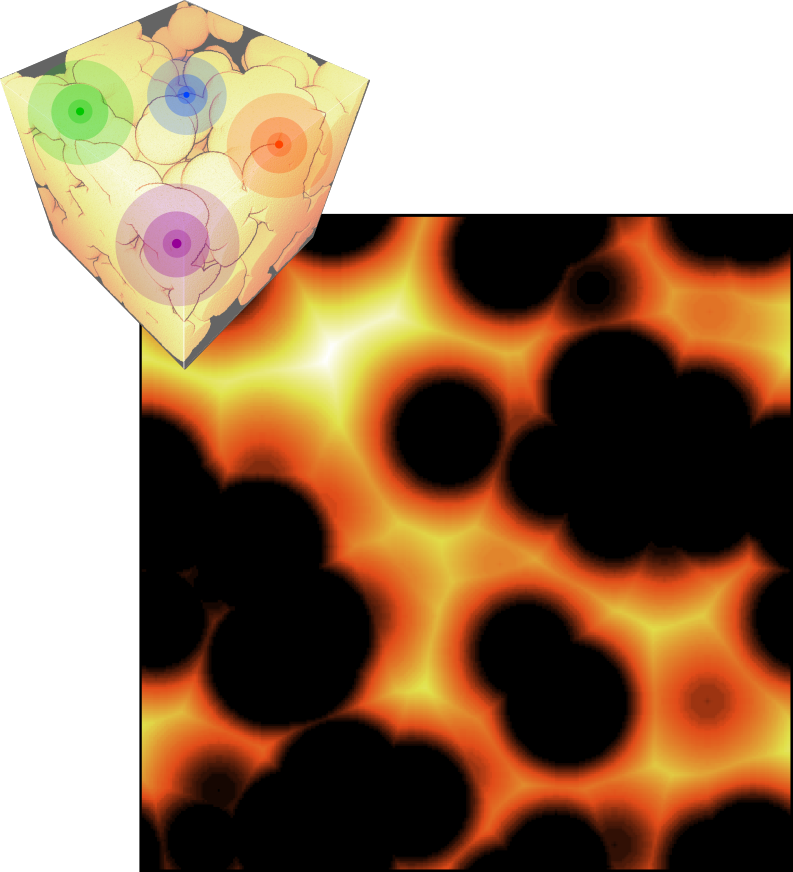• a binary 2D or 3D image, the white part represents the microstructure to analysis, OR a triphasic 2D or 3D image, with specific intensities: 0 for the surrounding void, meaningless, 255 for the solid phase, and the rest is considered as the porous phase.
The triphasic image can be the output of the plug-in "Extraction of porosity". This option is originally added to consider real images acquired with a specific device.

Optional input:
• the homotopic skeleton of the microstructure (the microstructure is loaded as an additional file).

Outputs:

• the H-tortuosity values, the H-scalarsas a function of the radius and the scale,
• the 2D or 3D map of the mean relative tortuosities.

Parameters:

• the sampling method choice (1D-sampling or stratified-sampling),
• the maximal distance value Dmax (tortuosity variations assessed from 1 to Dmax),
• the number of random points (N),
• a boolean for the use of the skeleton or not (if the skeleton is chosen, it has to be loaded by the 'Open' button, and the microstructure has to be loaded using the 'Select additional file' button (' ... ')).

The H-tortuosity-by-iterative-erosions is the computation of the H-tortuosity estimator of the microstructure, as seen by a spherical particle of given size. In addition to keeping the properties of the H-tortuosity, this descriptor is linked to the notion of constrictivity, characterizing the bottleneck effect.
The previous steps, defining the H-tortuosity, are applied on the microstructure, which is eroded step by step. The iterative erosions, using a unit sphere, allows to consider a large interval of radii, representing the size of the hypothetic percolating sphere.

### Deterministic M-tortuosity: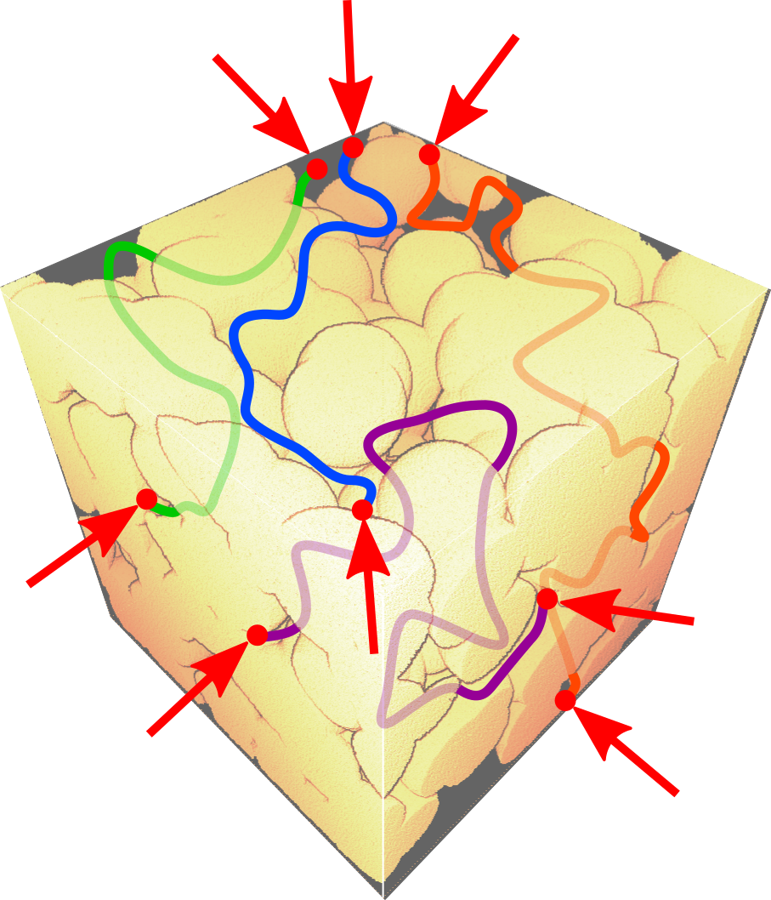Inputs:

• a binary 2D or 3D image, the white part represents the microstructure to analysis, OR a triphasic 2D or 3D image, with specific intensities: 0 for the surrounding void, meaningless, 255 for the solid phase, and the rest is considered as the porous phase.

The triphasic image can be the output of the plug-in "Extraction of porosity". This option is originally added to consider real images acquired with a specific device.

• a text file OR a binary 2D or 3D image, corresponding to the imposed locations, the given starting points.

Outputs:

• the deterministic M-tortuosity value, the M-scalar
• the set of M-coefficients
• the 2D or 3D map of the mean relative tortuosities.

Optional outputs:

• the pair "geodesic distance/morphological tortuosity" for each pair of starting points,
• the morphological tortuosities maps of each starting point,
• the geodesic paths for each pair of points (a map per starting point), combined or not to the corresponding morphological tortuosities map.

Parameters:

• the 'Select additional file' button (' ... ') for the imposed starting points, a text file (*.txt) OR an image (*.tif or *.fda)
• a boolean 'Image' to use an image for the definition of the given starting points (if an image is chosen, the boolean 'Image' has to be checked). Additional parameters (if checked):
1.     a boolean 'Center of Mass' (computation if checked): in the case of real particles as starting locations, the particles (objects bigger than 1 voxel) have to be reduced to a unique voxel, their center of mass,
2.   a boolean 'Orth. Projection' (computation if checked): due to some approximations, manual segmentation and/or center of mass computation, for instance, some starting points could no longer belong to the microstructure of interest. The orthogonal projection on the microstructure of interest can fix this problem by selecting the closest feature point (point inside the microstructure) to each starting point.

'Center of Mass' and 'Orth. Projection' can be used combined, first center of mass computation then orthogonal projection, or separately, center of mass or orthogonal projection.

• a boolean 'Save Dist/Tor' corresponding to an optional output; save all pairs "geodesic distance/morphological tortuosity" for each pair of starting points,
• a boolean 'Save Data' corresponding to the two other optional outputs. Additional parameters (if checked):
1.     a boolean 'Maps' (save if checked): the morphological tortuosities map of each starting point is saved as *.fda file,
2.     a boolean 'Paths' (save if checked): the geodesic paths of each starting points are saved as *.fda file,
3.     '...': selection of the save directory.

'Maps' and 'Paths' can be used combined, if this is the case, the geodesic paths are displayed over the morphological tortuosities maps, or separately, the morphological tortuosities maps or the geodesic paths.

The deterministic M-tortuosity is the computation of the M-tortuosity estimator of the microstructure, but with a given set of starting points. The properties of the M-tortuosity are kept. Moreover, real images can be now processed. To be more specific, images acquired with electron tomography can be considered as triphasic images: the porous volume, the solid phase, and the meaningless surrounding void.

### M-tortuosity:Input:

• a binary 2D or 3D image, the white part represents the microstructure to analysis, OR a triphasic 2D or 3D image, with specific intensities: 0 for the surrounding void, meaningless, 255 for the solid phase, and the rest is considered as the porous phase.
The triphasic image can be the output of the plug-in "Extraction of porosity". This option is originally added to consider real images acquired with a specific device.

Outputs:

• the M-tortuosity value, the M-scalar
• the set of M-coefficients
• the 2D or 3D map of the mean relative tortuosities.

Optional outputs:

• the pair "geodesic distance/morphological tortuosity" for each pair of starting points,
• the morphological tortuosities maps of each starting point,
• the geodesic paths for each pair of points (a map per starting point), combined or not to the corresponding morphological tortuosities map.

Parameters:

• the sampling method choice (1D-sampling or stratified-sampling),
• the number of random points (N),
• a boolean 'Save Dist/Tor' corresponding to an optional output; save all pairs "geodesic distance/morphological tortuosity" for each pair of starting points,
• a boolean 'Save Data' corresponding to the two other optional outputs. Additional parameters (if checked):
1.     a boolean 'Maps' (save if checked): the morphological tortuosities map of each starting point is saved as *.fda file,
2.     a boolean 'Paths' (save if checked): the geodesic paths of each starting points are saved as *.fda file,
3.     '...': selection of the save directory.

'Maps' and 'Paths' can be used combined, if this is the case, the geodesic paths are displayed over the morphological tortuosities maps, or separately, the morphological tortuosities maps or the geodesic paths.

Computation of the M-tortuosity estimator: a scalable topological descriptor providing a 3D map of mean relative tortuosities, a set of M-coefficients, of which the histogram is meaningful, a final scalar value, the M-tortuosity value, named the M-scalar, assessing the morphological tortuosity of the overall microstructure.
The descriptor, based on a Monte Carlo process, handles disconnections and is applicable on complex multi-scale microstructures, especially when entries and exits are delicate to imposed. Moreover, real images can be now processed. To be more specific, images acquired with electron tomography can be considered as triphasic images: the porous volume, the solid phase, and the surrounding void. This last phase is meaningless. The various steps and associated parameters are exposed below.

1.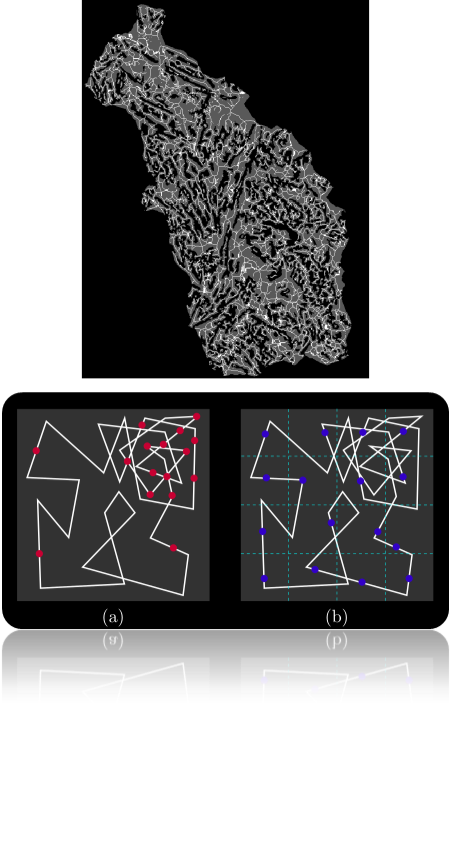Optional step: an optional step is the skeletonization. We recommand the computation of an homotopic skeleton of the interconnected microstructure to analysis. The homotopic skeleton is theoretically the smallest subset of the microstructure keeping the connectivity degree. In other words, the topology is unchanged. An exemple is shown in right figure.
Various computational methods are proposed in the state-of-the-art. Plug-ins will be available for the skeletonization step, different algorithms will be proposed according to the application, i.e. the nature of the image.

2. Sampling: The first step is the sampling of a chosen number of points N. Either N is the exact number of sampled points, if the 1D-sampling method (a) is chosen, or N is an approximated value, i.e. a target value, if the stratified-sampling method (b) is chosen. The next figure displays the difference the two sampling methods (a) and (b).

3. Distance transforms computation: The distance transforms and the geodesic distance transforms are computed. At this step, the 2D or 3D map of mean relative tortuosities is assessed.

4. M-coefficients: The M-coefficients can be seen as a set of morphological tortuosities, each one according to a specific random point, in other words " a viewpoint " of the microstructure of interest. A M-coefficient, connected to a specific location, is the assessed tortuosity at this point. It can be seen as either a viewpoint of the microstructure from this location or the average accessibility of this point for any random point of the microstructure.

5. M-scalar: The M-tortuosity value, named the M-scalar, is a " global viewpoint ". It aggregates information of each viewpoint, the M-coefficients, into a unique scalar value.

### M-tortuosity-by-iterative-erosions: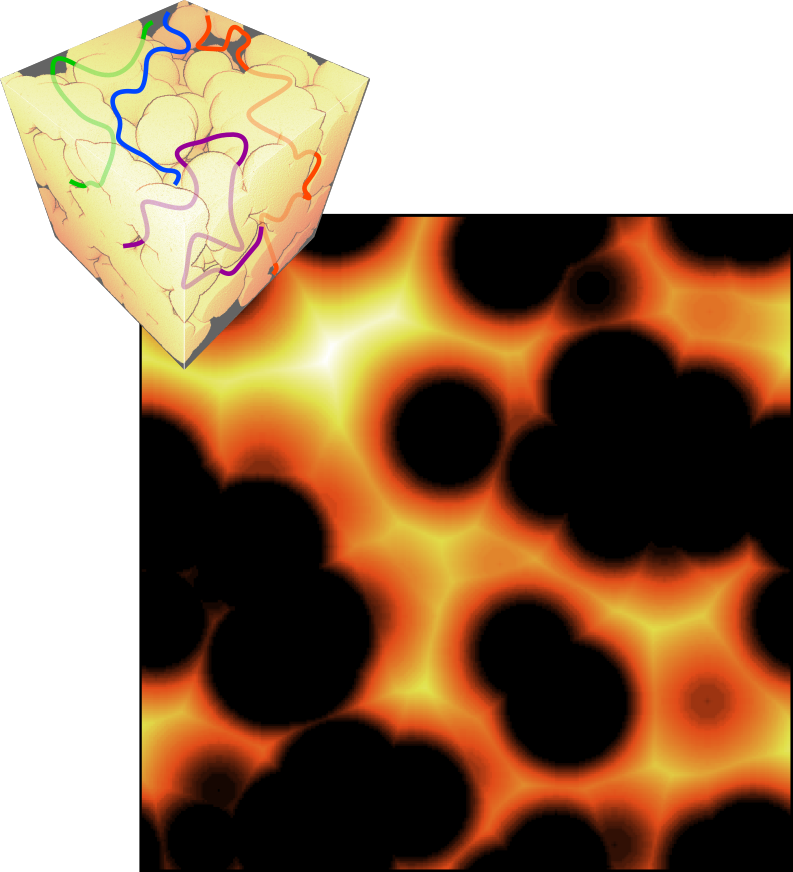Input:

• a binary 2D or 3D image, the white part represents the microstructure to analysis, OR a triphasic 2D or 3D image, with specific intensities: 0 for the surrounding void, meaningless, 255 for the solid phase, and the rest is considered as the porous phase.
The triphasic image can be the output of the plug-in "Extraction of porosity". This option is originally added to consider real images acquired with a specific device.

Optional input:

• the homotopic skeleton of the microstructure (the microstructure is loaded as an additional file).

Outputs:

• the M-tortuosity values, the M-scalarsas a function of the radius,
• the set of set of M-coefficientsas a function of the radius,
• the 2D or 3D map of the mean relative tortuosities.

Parameters:

• the sampling method choice (1D-sampling or stratified-sampling),
• the number of random points (N),
• a boolean for the use of the skeleton or not (if the skeleton is chosen, it has to be loaded by the 'Open' button, and the microstructure has to be loaded using the 'Select additional file' button (' ... ')).

The M-tortuosity-by-iterative-erosions is the computation of the M-tortuosity estimator of the microstructure, as seen by a spherical particle of given size. In addition to keeping the properties of the M-tortuosity, this descriptor is linked to the notion of constrictivity, characterizing the bottleneck effect. Moreover, real images can be now processed. To be more specific, images acquired with electron tomography can be considered as triphasic images: the porous volume, the solid phase, and the surrounding void. This last phase is meaningless.
The previous steps, defining the M-tortuosity, are applied on the microstructure, which is eroded step by step. The iterative erosions, using a unit sphere, allows to consider a large interval of radii, representing the size of the hypothetic percolating sphere.

The homotopic skeleton allows a consistent reducing of the computation time. Indeed, the biggest part of the total computation time is taken by the computation of the geodesic distance maps. For a geodesic distance map, and a constant complexity, the more feature points, the longer the computation time.
Moreover, when the homotopic skeleton is selected, the computation time of the morphological erosion is reduced too. The morphological erosion is efficiently computed using distance transform. The eroded set of an original set, is assessed by applying a threshold over the distance map of the original set. Instead of computing a distance map at each step, we keep the original distance map and increase the threshold, at each step of the algorithm. The skeleton is used to compute the valued skeleton; i.e. at each location of a skeleton point, the distance map value is assigned. This means less points to check, less wasting time.

.